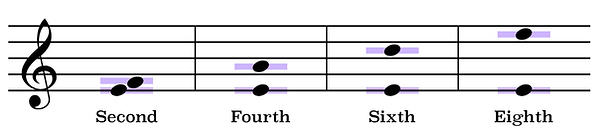top of page

## Interval Identification | Tips And Tricks (10 of 10)

When reading intervals on the staff it is helpful to know that with each of the even numbered intervals, such as Major and minor seconds, Perfect fourths, Major and minor sixths, as well as Perfect eighths (or octaves) if the bottom note of the interval is on a line, then the top note will always be on a space.Likewise, if the bottom note of an even numbered interval is on a space, then the top note will always be on a line.On the other hand, the notes of an odd numbered interval such as Major and minor thirds, Perfect fifths, as well as Major and minor sevenths will either both be on a spaceor both be on a line.The ability to recognize odd and even numbered intervals based solely upon each note's position on the staff will allow you to determine the identity of each interval much more quickly and efficiently.

For example, without actually knowing the letter name of these two notes, just by seeing that the bottom note is on a space while the top note is on a line, knowing that even numbered intervals are always on opposite staff positions, I can tell that the distance separating these two notes is in fact an even numbered interval.Now, seeing how these notes are too far apart to be an interval of a second, and probably too close together to be an interval of a sixth, or an octave, by process of elimination I can safely assume that the distance separating each note is in fact an interval of a fourth.Seeing how the notes in this next example are both on a line, I know that the distance between them must be an odd numbered interval.Without actually counting the steps, we can see that these notes are definitely too far apart to be an interval of a third, and are probably too far apart to be an interval of a fifth as well. Therefore, the amount of steps between them must then be that of an interval of a seventh.In the first video of this series we learned that intervals can either be played as ascending - in other words, from the note with the lower pitch frequency to the note with the higher pitch frequency, or as descending - from the higher note to the lower note.

Some people find that reading descending intervals on the staff can be a bit confusing, especially when accidentals are involved.

Take for example the distance between the note E-flat and the first G below it.An easy way to discover what interval separates these two notes would be to simply read them in reverse.

By that I mean, rather than seeing this as E-flat descending to G, temporarily look at it as being the note G ascending to the note E-flat.By seeing it in this way it becomes easier to determine that they are separated by an interval of a minor sixth.

As you can see, just by reading these notes in reverse we did not alter the amount of steps between them. Therefore, the distance from E-flat descending to the note G is also an interval of a minor sixth.This technique may be applied to discover any descending interval.

## Difference Between Major And Minor Intervals

You may have noticed throughout this video series that minor intervals are always one half step lower than their Major counterparts.

In the third video of this series in which we discussed intervals of a third we learned that an interval of a minor third occurs when two notes are separated by the distance of three consecutive half steps, while an interval of a Major third occurs when two notes are separated by the distance of four consecutive half steps.

Therefore, knowing that an interval of a Major third above the note C is the note E,even without counting steps, we can safely assume that an interval of a minor third above the note C is the note E-flat.Likewise, if an interval of a Major seventh above the note G is the note F#then an interval of a minor seventh above G is F natural.bottom of page